# Hours

The lesson lasts 45 minutes. For the week, students have 18 lesson hours. How many are the actual hours?

Correct result:

n =  13.5 h

#### Solution: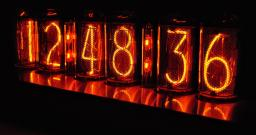We would be pleased if you find an error in the word problem, spelling mistakes, or inaccuracies and send it to us. Thank you!Tips to related online calculators
Do you want to convert time units like minutes to seconds?

## Next similar math problems:

• Nightingale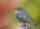Nightingale sang five seconds for 5 hours and 5 minutes. How many seconds were missing to sing 10 hours?
• Time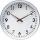16.2 days ..... how many hours is it?
• Water tankIn an empty fire tank, 2150 hl of water jetted in 5 hours. How many hectoliters of water was jetted every hour? How many hectoliters of water was in the tank after three hours?
• Hr to minSue biked to school in 5/12 of an hour. How many minutes did it take her to ride to school?
• Hours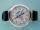How many hours is 9 days?
• Clock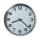How many hours are 15 days?
• Pump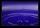From the pump in the yard dripping water. Every drop is 1 ml. How many drops will be necessary before filling a bucket with a volume of 10 l? Calculate how long it will fulfill the bucket when drops fall each second.
• Bean bag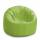A student tossed a bean bag. It landed 216 inches away. How many yards are equal to 216 inches?
• Illness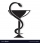Peter is ill and must take medication three times a day for one tablet. For how many days is a pack of 30 tablets enough for him, when on the third day he takes one tablet only in the morning and evening?
• Father and son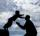Father is 44 years old, his son 16 years. Determine how many years ago was the father five times older than the son.
• Minutes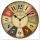Determine the difference in minutes: T1 = 2 3/20 h T2 = 2.3 h
• Math classificationIn 3A class are 27 students. One-third got a B in math and the rest got A. How many students received a B in math?
• The number 3Ski organizers should print the start numbers from 1 to 45. How many times will they use the number 3 when printing?
• The resultHow many times I decrease the number 1632 to get the result 24?
• Circles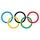For the circle c1(S1; r1=146 cm) and c2(S2; r2 = 144 cm) is distance of centers |S1S2| = 295 cm. Determine the distance between the circles.
• Eq1Solve equation: 4(a-3)=3(2a-5)Added together and write as decimal number: LXVII + MLXIV